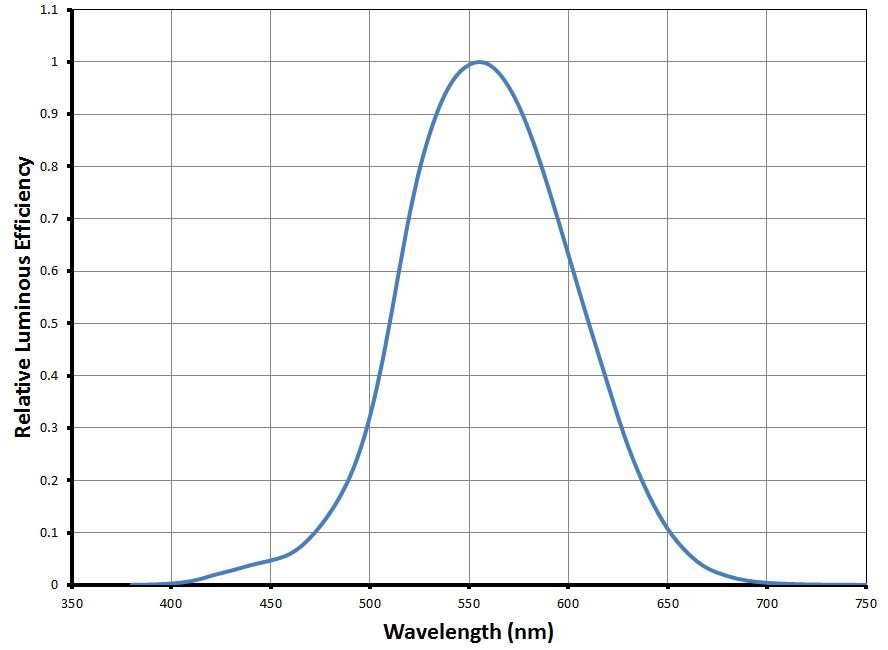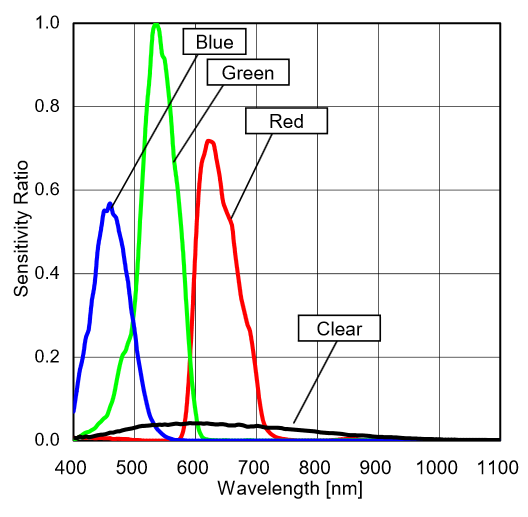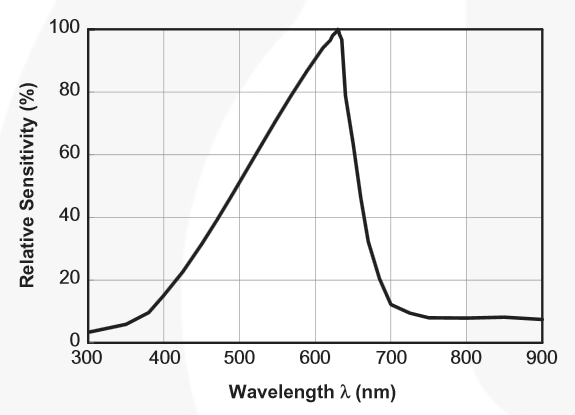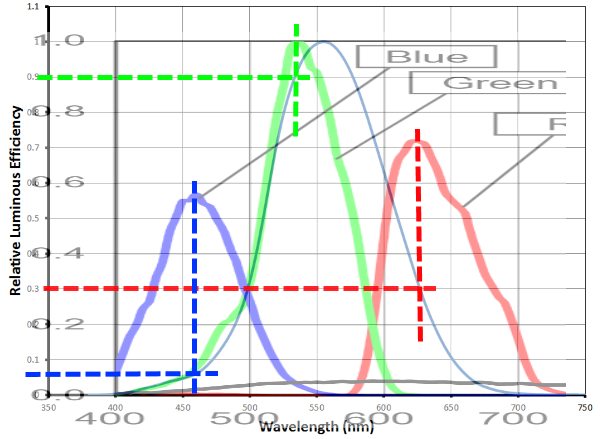Technical Article

# Measuring and Calculating Lux Values, Part 2

January 15, 2016 by Robert Keim

## Use data from an RGB sensor to improve the accuracy of illuminance measurements.

Use data from an RGB sensor to improve the accuracy of illuminance measurements.

### A Quick Review

In the previous article we covered the relationship between irradiance and illuminance in the context of light-sensitive electronic components. The first factor governing this conversion is the optical sensor’s sensitivity, i.e., the relationship between light intensity and output current (or digital counts, if the sensor is an IC that processes the raw sensor signal into digital information). The second factor—actually, a continuous function of factors—is the number, based on the spectral sensitivity of the human eye, that we use to convert irradiance (in watts per square meter) to illuminance (in lux). This number is 683 for electromagnetic radiation at 555 nm, and it decreases as follows for higher or lower wavelengths:One way to measure illuminance, as discussed in the preceding article, is to use an optical sensor that is designed to approximate the spectral response of human vision. The datasheets for these devices usually have information that allows you to convert directly from output to lux; however, owing to the discrepancy between the ideal spectral response (i.e., the luminosity function) and the sensor’s spectral response, the accuracy of the measurement will vary depending on the spectral composition of the ambient illumination.

### The RGB Approximation

A highly accurate illuminance measurement could be performed as follows: first, you need an optical sensor with a “flat” spectral response—i.e., the sensitivity is the same for every wavelength in the visible spectrum. Call this the wideband detector. In addition, you arrange in close proximity numerous narrowband detectors fine-tuned to a particular wavelength. The wideband detector produces an output proportional to the overall light intensity, with each wavelength contributing equally, and the narrowband detectors reveal the spectral composition of the light. You can then apply the luminosity function, based on this spectral information, to the output of the wideband detector.

The precision achieved with this approach will be proportional to the number of narrowband detectors—more detectors means a more accurate representation of the actual spectral composition. Practical limitations quickly come into play, though, and furthermore—as discussed in the first article—highly precise illuminance measurements are unnecessary and, in a certain sense, impossible.

Thus, we can implement this approach but in a highly simplified form: we need a wideband detector with a fairly flat spectral response and three narrowband detectors. The obvious choice for the narrowband detectors are red, green, and blue—first, because RGB sensors are readily available, and second, because the RGB wavelengths divide the visible spectrum into three more or less equal portions:This is the spectral sensitivity information for the Rohm RGBC sensor IC (p/n BH1745NUC) mentioned in the previous article. As you can see, the blue detector corresponds to about the lower one-third of the visible spectrum, the green detector corresponds to the middle one-third, and the red detector corresponds to the upper one-third. Note also that the response of the clear detector is fairly flat—actually, it is very flat compared to the curves presented in the previous article, such as this one for the Fairchild phototransistor (p/n KDT00030TR) :Nevertheless, the response of the Rohm part drops significantly at the lower wavelengths . . . I fully understand the difficulty this causes for those inclined to perfectionism—but remember, low-cost, low-complexity illuminance measurements are not an exercise in precision!

### Step by Step

Let’s go through the process of calculating a lux measurement using the BH1745NUC. One piece of information we will need is the sensitivity of the clear detector, which we calculated in the preceding article as

$\frac{160\ counts}{0.2\ \frac{W}{m^2}}=\frac{800\ counts}{\frac{W}{m^2}}=\frac{1\ count}{0.00125\frac{W}{m^2}}$

1. We need to adjust the RGB measurements to compensate for the major differences in sensitivity. From the above plot we see that R is about 0.72 and B is about 0.56 when G is 1. Thus, we multiply the R and B values by the appropriate correction factor:

$CF_R=\frac{1}{0.72}=1.39,\ \ \ CF_B=\frac{1}{0.56}=1.79$

2. Determine the “portion” of the ambient light contained in the R, G, and B bands by adding up the three values and dividing each by the total. This approach presupposes the assumption whereby the R, G, and B values represent all the irradiance in the upper, middle, and lower third of the visible spectrum. For example, let’s say the corrected RGB outputs are as follows:

$R=75\ counts,\ \ G=100\ counts,\ \ B=75\ counts$

The “irradiance portion” (IP) for each color is thus

$IP_R=\frac{75}{250}=30\%,\ \ IP_G=\frac{100}{250}=40\%,\ \ IP_B=\frac{75}{250}=30\%$

3. Find the irradiance-to-illuminance conversion factor for each color:From this overlay plot we can estimate the following irradiance-to-illuminance factors, recalling that the maximum at 555 nm is 683:

$IRtoIL_R=683\times0.3=205,\ \ IRtoIL_G=683\times0.9=615,\ \ IRtoIL_B=683\times0.06=41$

4. Multiply the irradiance portions by the appropriate IRtoIL factor, and add up the results to determine the overall IRtoIL factor for the particular spectral composition of the currently measured illumination:

$IRtoIL_{overall}=\left(205\times0.3\right)+\left(615\times0.4\right)+\left(41\times0.3\right)=320$

5. Use the clear detector’s sensitivity to determine the wavelength-independent irradiance. Let’s say the clear output is 300 counts:

$300\ counts\div\frac{800\ counts}{\frac{W}{m^2}}=0.375\ \frac{W}{m^2}$

$0.375\ \frac{W}{m^2}\times320=120\ lux$

### Discussion and Conclusion

If you read through this process a few times, I think you will find that it is quite intuitive. One way to think about the fundamental concept is the following: if the clear detector were illuminated by a pure red light and then a pure green light of the same intensity, the output would not change (assuming that the clear detector is equally sensitive to all wavelengths). This means that at least one of the corresponding lux values cannot be anywhere near correct, because the irradiance-to-illuminance factor for red is much lower than for green—in this case, 205 vs. 615. So what we are doing here is finding the “average” conversion factor based on how much R, G, and B radiation is in the ambient light. If the light were pure red, IRR would be 100%, and IPG and IPB would be 0%. Thus, the overall conversion factor would be the same as the conversion factor for red, i.e., 205. If the light were half pure red and half pure green, the overall conversion factor would be the average of the red and green conversion factors, i.e., (205 + 615)/2 = 410.

You might be wondering if the clear detector is really necessary here. That’s a difficult question. We could ignore the clear detector and simply interpret the sum of the three RGB measurements as the total irradiance, but we would end up ignoring irradiance that falls in the wavelengths not covered by the RGB sensitivity bands. However, if the wavelengths of this ignored irradiance are toward the edges of the luminosity function, it would actually be better to ignore it—if the RGB detectors don’t see it and the clear detector does, it will make an erroneously large contribution to the final lux value. I suppose the bottom line is that both approaches are feasible, with one or the other being more accurate depending on the spectral characteristics of the lighting conditions and of the clear detector—ignoring the clear detector is an easy way to eliminate error caused by its nonideal (i.e., non-wavelength-independent) sensitivity.

The procedure described here is perhaps more of a starting point. It should provide reasonably accurate illuminance measurements with minimal investment of time and money, but you should definitely consider ways to refine your algorithm based on empirical observations or, even better, by comparing your results to those of a high-quality lux meter.